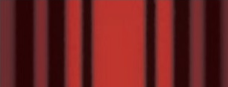# Problem: (Figure 1) shows the light intensity on a viewing screen behind a single slit of width a. The light's wavelength is λ.Is λ &lt; a, λ = a, λ &gt; a, or is it not possible to tell? Answer the question by filling in the terms or equations to the appropriate blanks to complete the sentences.(a) The width of the central maximum in the intensity pattern for a single slit is given by ___________.(b) If λ ≥ a than w will be equal to or ________ 2L.(c) It is obvious that w &lt;&lt; 2L, hence λ is _______ a.w = 2Lλ/aw = 2aL/λw = 2La/λgreater thanless thanequal tow = 2aλ/L

###### FREE Expert Solution

(a)

Width of central maximum for single slit:

$\overline{){\mathbf{w}}{\mathbf{=}}\frac{\mathbf{2}\mathbf{L}\mathbf{\lambda }}{\mathbf{a}}}$

The width of the central maximum in the intensity pattern for a single slit is given by 1. w = 2Lλ/a

100% (62 ratings)###### Problem Details

(Figure 1) shows the light intensity on a viewing screen behind a single slit of width a.The light's wavelength is λ.

Is λ < a, λ = a, λ > a, or is it not possible to tell?

Answer the question by filling in the terms or equations to the appropriate blanks to complete the sentences.

(a) The width of the central maximum in the intensity pattern for a single slit is given by ___________.

(b) If λ ≥ a than w will be equal to or ________ 2L.

(c) It is obvious that w << 2L, hence λ is _______ a.

1. w = 2Lλ/a
2. w = 2aL/λ
3. w = 2La/λ
4. greater than
5. less than
6. equal to
7. w = 2aλ/L